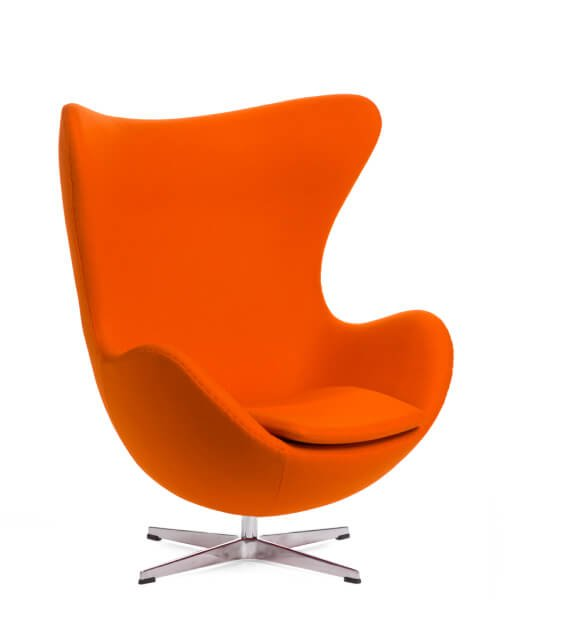•en

# What is Topology?

In three-dimensional (3D) visualization, topology is used to describe the way surfaces are connected to each other. A surface can be thought of as a collection of points, called vertices, and the lines connecting those points, called edges. The way in which the vertices and edges are connected together defines the topology of the surface. The most common topologies in 3D visualization are called triangle strips and quad strips.

• In a triangle strip, the vertices are connected together in a triangular pattern. Each vertex is connected to the two vertices that are next to it in the strip. This type of topology is often used for modeling objects such as curved surfaces.
• In a quad strip, the vertices are connected together in a rectangular pattern. Each vertex is connected to the four vertices that are next to it in the strip. This type of topology is often used for modeling objects such as flat surfaces.

Topology is also used to describe the way volumes are connected to each other. A volume can be thought of as a collection of points, called voxels, and the cubes connecting those points, called faces. The way in which the voxels and faces are connected together defines the topology of the volume. The most common topology in 3D visualization is called a hexahedral mesh.Fill out the form
We will reply within 1 working day